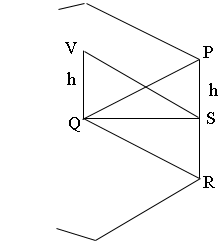SEARCH HOMEMath Central Quandaries & QueriesSubject: solid geometry Name: wendimu Who are you: Student The altitude of regular n-side pyramid is half of it's base side.What is the angle between the lateral face and it's base?Wendimu,

Wow! The answer to this question is really nice. In my diagram PR is a side of the regular n-sided polygon that forms the base of the pyramid and Q is the centre of the polygon. V is the vertex of the pyramid and S is the midpoint of PR.I let h be the height of the pyramid and hence |PS| = h. Triangles PQS and VQS are right triangle with a common base QS and the same height h. Thus triangles PQS and VQS are congruent. Hence the angle QSV, which is the angle between the lateral face and the base, is congruent to the angle PQS. What is the measure of the angle PQS?

PennyMath Central is supported by the University of Regina and The Pacific Institute for the Mathematical Sciences.